Music and Geometry 2: Pitch Class Circles and Composing

In the previous article we introduced the concept of pitch and pitch class. We saw how notes could be represented on a pitch line, forming a one-dimensional geometrical space, and how removing octave information caused the line to turn into a circle.

The pitch class circle, despite first appearance, is still a one-dimensional space (but it’s a curved one). The reason is that, like on the pitch line, you still need only one number (a so-called coordinate) to unambiguously address a point on the circle compared to a chosen reference point. We’ll explore this idea and some of its consequences later in this article. We will look at some properties and applications of the pitch class circle, getting a first taste of how strange life in curved spaces can be.

Composition operations on the pitch class circle

Geometrical operations

As with the pitch line, the pitch class circle allows to perform certain composition operations.

Transposition of pitch classes is done by traveling along the pitch class circle. After the transposition is done, the composer is free to assign octaves to convert from pitch class back to pitch.

Both example 1 and 2 in the following picture can be represented on the pitch class circle below.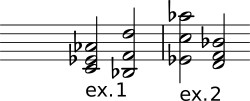Inversion mirrors around a diameter of the circle: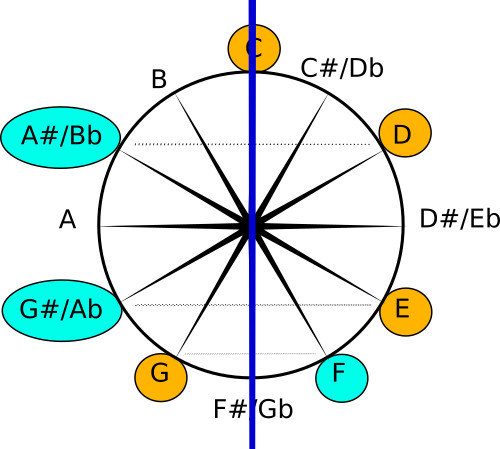Mathematical equivalent of geometric operations

Instead of drawing pitch class circles, and sliding or mirroring pitches, one can also do something easier. Remember how I said that the pitch class circle is a one-dimensional space? And we needed only one number (a coordinate) to unambiguously name any pitch on the circle? Well, let’s choose the “C” class as reference (i.e. we give it the coordinate 0) and assign a coordinate to the remaining pitches. Note that with our choice of numbers, microtonal pitches can be represented as floating point numbers. E.g. F quarter sharp would have a coordinate 5.5, because it falls exactly between F (with coordinate 5) and F# (with coordinate 6).

Note that coordinate 12 does not occur on the pitch class circle. Coordinate 12 is equivalent to coordinate 0 on the pitch class circle. Whenever we do mathematics operations while working with pitch classes and we end up with numbers >= 12, we need to keep subtracting 12 until we end up lower than 12 again. In math speak, we say that we calculate “modulo 12”, and that “12 is congruent with 0 modulo 12”.

When you calculate modulo 12, you can write funny sums like: 11 + 3 = 2 (mod 12). Why? Well, 11+3 in the normal universe equals 14, but we’re calculating modulo 12, so we need to subtract 12 until the result is smaller than 12, and we get 14-12=2. Life in curved spaces at first sight seems different from what we’re used to in everyday life but attentive readers probably have noticed that clock reading uses the exact same form of modulo 12 arithmetic. 3 hours later than 11 o’clock indeed is 2 o’clock. Perhaps this is the reason that in at least one other exotic music theory I’m aware of the pitch class circle is also known as a tone clock.Using the coordinates we can now also do the same composition operations we did using geometry before using only purely mathematical operations. Transposition boils down to adding a constant number to each note-coordinate.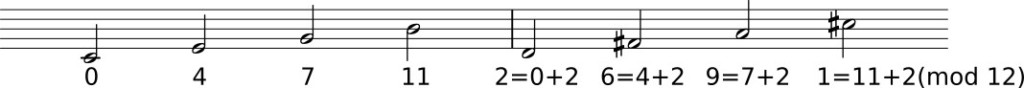Inversion boils down to subtracting the note-coordinate from the constant number 12.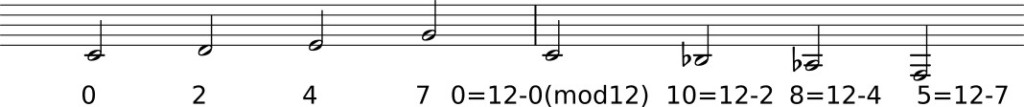Beyond geometry

Now that we can use numbers instead of geometry, nothing stops us from performing other operations on pitches and pitch classes. What happens to a melody if you multiply all pitch coordinates with 2? What happens if you take your 2 favorite melodies and calculate their average? What happens if you take two melodies and you multiply them pitch by pitch? Your imagination and curiosity are the only limits here. Let’s see some simple examples.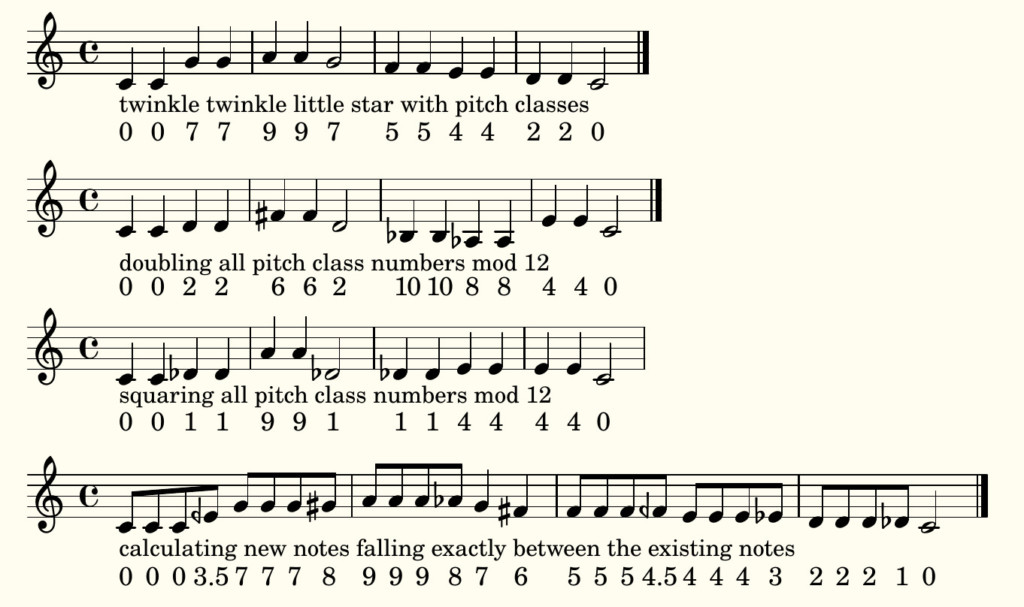Listen to the different fragments here:

Combining two different melodiesListen to the different fragments here:

PETER’S WOLF’S WEIRD WALK: AVERAGED

Finally, let’s listen to all fragments simultaneously (just for fun!).

TUTTI

Note that on the pitch line you can also assign numbers to notes and do calculations but there the value of C4 and C5 will differ 12. There’s no modulo calculation there (no curved space and no octave equivalence)

Using the pitch class circle to analyze scale symmetry

Neither the pitch line nor the pitch class circle in themselves are the right or wrong representation. The pitch class circle offers possibilities
for analysis and composition that were not readily present in the pitch line representation.

As an example, the pitch class circle immediately and very visually shows you if a collection of notes belong to a symmetrical scale. This is illustrated in the following picture, where some important symmetrical scales/modes are illustrated (there are many more). The symmetry is visually present.

There are more music-theoretical things one can do with the pitch class circle, but in this article I won’t go any deeper.In this article we saw how pitch classes can be assigned numbers, how we can calculate with these numbers. We saw how closely connected the concepts of musical pitch class, curved spaces and modular arithmetic are connected.

In the next articles we will investigate “intervals” instead of “pitches”. We will leave our one-dimensional spaces (pitch line and pitch class circle) behind, and will end up exploring two-dimensional curved spaces, where life is even more strange and fascinating.

Scroll to Top